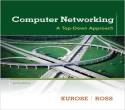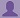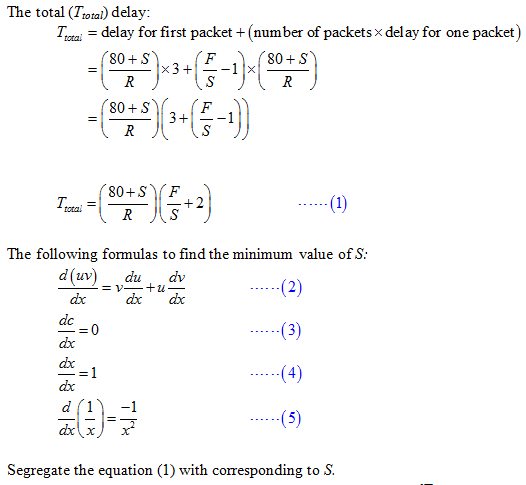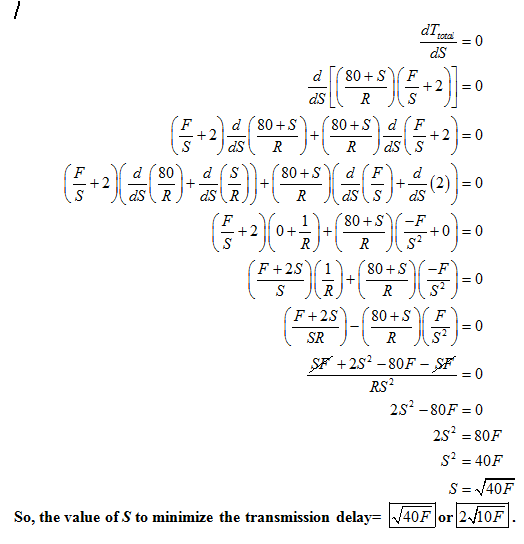SHARE
HELP

To start with, Sr2Jr’s first step is to reduce the expenses related to education. To achieve this goal Sr2Jr organized the textbook’s question and answers. Sr2Jr is community based and need your support to fill the question and answers. The question and answers posted will be available free of cost to all.# Computer Networking : A Top-down Approach

Authors:
James F. Kurose, Keith W. Ross
Exercise:
Problems
Chapter:
Computer Networks And The Internet
Edition:
6
ISBN:
9780132856201
Question:
34
 Previous Next

###Question

Consider sending a large file of bits from Host Ato Host B. There are three links (and two switches) between Aand B, and the links are uncongested (that is, no queuing delays). Host Asegments the file into segments of bits each and adds 80 bits of header to each segment, forming packets of = 80 + bits. Each link has a transmission rate of bps. Find the value of that minimizes the delay of moving the file from Host Ato Host B. Disregard propagation delay.

###Answer

Consider the given data:

Number of switches=2

File size=F bitsze of each Segment= S bits

Number of segments=F/S

Packet size(L)=80+S bits

Transmission rate(R)=R bps

Transmission delay(Tdelay)=L/R

=(80+S)/R  seconds

Time (T) required for the first packet to be transmitted to destination:

=(80+S)/R *3### Discussions

Post the discussion to improve the above solution.